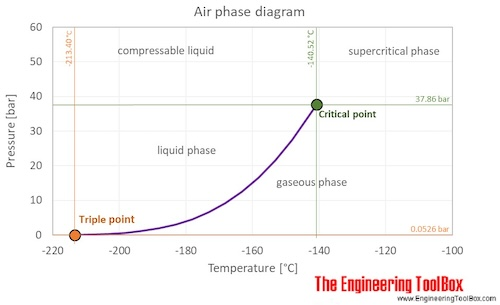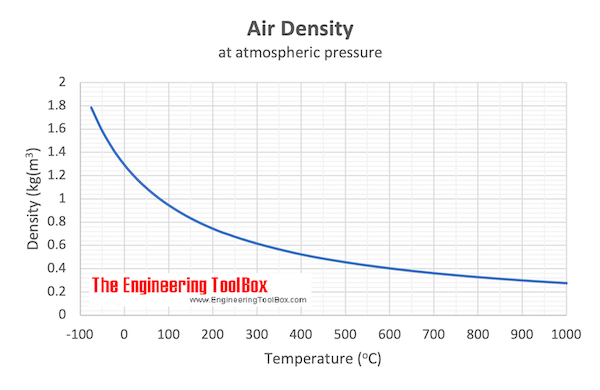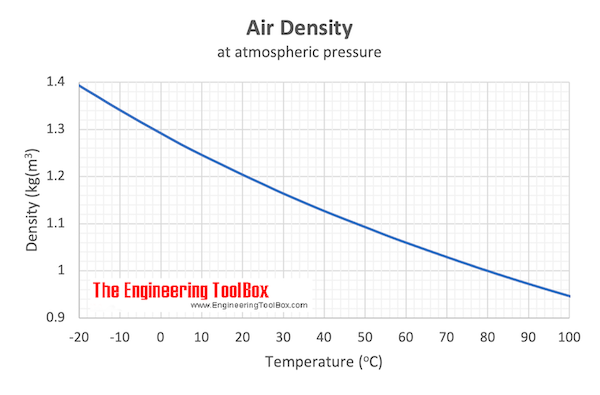Engineering ToolBox - Resources, Tools and Basic Information for Engineering and Design of Technical Applications!

# Air - Thermophysical Properties

## Thermal properties of air at different temperatures - density, viscosity, critical temperature and pressure, triple point, enthalpi and entropi, thermal conductivity and diffusivity and more.

Thermophysical properties of air:

• Boiling temperature (at 1 bara):                            78.8 K = -194.4 °C = -317.8 °F
• Bulk modulus elasticity :                                         1.01325 x 10 5 Pa or N/m 2
• Condensation temperature (at 1 bara):                 81.8 K = -191.4 °C = -312.5 °F
• Critical temperature :                                              132.63 K = -140.52 °C = -220.94 °F
• Critical pressure :                                                    37.363 atm = 37.858 bar = 3.7858 MPa (MN/m 2 ) = 549.08 psi (=lb f /in 2 )
• Critical density:                                                      10.448 mol/dm 3 = 302.6 kg/m 3 = 0.5871 slug/ft 3 = 18.89 lb m /ft 3
• Density (at 0°C and 1 bara):                                 1.276 kg/m 3 = 0.00248 slug/ft 3 = 0.0797 lb/ft 3
• Density (at 60°F and 1 atm):                                  1.208 kg/m 3 = 0.00234 slug/ft 3 = 0.0754 lb/ft 3
• Enthalpy (heat) of air at 0°C  and 1 bara:              11.57  kJ/mol = 399.4  kJ/kg = 171.7 Btu(IT)/lb
• Entropy of air at 0°C  and 1 bara:                           0.1100 kJ/mol K = 3.796 kJ/kg K = 0.9067 Btu(IT)/lb °F
• Liquid density at boiling point and 1 bar:                875.50 kg/m 3 = 54.656 lb/ft 3
• Molar mass: 28.9647 g/mol
• Specific heat capacity (C p ) air at 0°C and 1 bara:  1.006 kJ/kgK = 0.24028 Btu(IT)/(lb m °F) or kcal/(kg K)
• Specific heat capacity (C v ) air at 0°C and 1 bara:  0.7171 kJ/kgK = 0.17128 Btu(IT)/(lb m °F) or kcal/(kg K)
• Thermal conductivity at 0°C and 1 bara:                 24.35 mW/(m K) = 0.02094 kcal(IT)/(h m K) = 0.01407 Btu(IT)/(h ft °F)
• Thermal expansion coefficient at 0°C and 1 bara:  0.00369 1/K  = 0.00205 1/°F
• Triple point pressure:                                              0.05196 atm = 0.05265 bar = 5265 Pa = 0.7636 psi (=lb f /in 2 )
• Triple point temperature:                                         59.75 K = -213.40 °C = -352.12 °F
• Viscosity, dynamic , at 0°C and 1 bara:                    17.22 μPa s = 0.01722 cP = 0.3596x 10 -6 ( lb f s)/ft 2 = 11.57 x10 -6 lb m /(ft s)
• Viscosity, kinematic , at 0°C and 1 bara:                   0.00001349 m 2 /s = 13.49 cSt = 0.0001452 ft 2 /s

Follow the links below to get values for the listed properties of air at varying pressure and temperature :

See also more about atmospheric pressure , and STP - Standard Temperature and Pressure & NTP - Normal Temperature and Pressure ,
as well as Thermophysical properties of: Acetone , Acetylene , Ammonia , Argon , Benzene , Butane , Carbon dioxide , Carbon monoxide , Ethane , Ethanol , Ethylene , Helium , Hydrogen , Hydrogen sulfide , Methane , Methanol , Nitrogen , Oxygen , Pentane , Propane , Toluene , Water and Heavy water, D 2 O .

Air is a mixture of gases at standard conditions. However, at low temperature and high pressures the gas mixture becomes a liquid. The phase diagram for air shows the phase behavior with changes in temperature and pressure. The curve between the triple point and the critical point shows the air boiling point with changes in pressure.At the critical point there is no change of state when pressure is increased or if heat is added.

The triple point of a substance is the temperature and pressure at which the three phases (gas, liquid, and solid) of that substance coexist in thermodynamic equilibrium.Example - Mass of Air at Temperature 100 o C

From the table above - the density of air is 0.946 kg/m 3 at 100 o C . The mass of 10 m 3 air can be calculated as

m = V ρ

= (10 m 3 ) (0.946 kg/m 3 )

= 9.46 kg

where

m = mass (kg)

V = volume (m 3 )

ρ = density (kg/m 3 )

Example - Mass of Air at Temperature 20 o C

From the table above - the density of air is 1.205 kg/m 3 at 20 o C . The mass of 10 m 3 air can be calculated as

m = (10 m 3 ) (1.205 kg/m 3 )

= 12.05 kg

Example - Lifting Force of a Hot Air Balloon

An air balloon with volume 10 m 3 is heated to 100 o C . The temperature of the surrounding air is 20 o C. The change in gravity force (weight) of the air volume is the potential lifting force of the balloon. The lifting force can be calculated as

F l = dm a g

= V d ρ a g

= (10 m 3 ) [(1.205 kg/m 3 ) - (0.946 kg/m 3 )] (9.81 m/s 2 )

= 25.4 N

where

F l = lifting force - change in gravity force (weight)  (N)

a g = acceleration of gravity (9.81 m/s 2 )

dm = V d ρ = change of mass in the balloon (kg)

dρ = change in density due to temperature difference (kg/m 3 )

## Related Topics

• ### Air Psychrometrics

Moist and humid air - psychrometric charts, Mollier diagrams, air-condition temperatures and absolute and relative humidity and moisture content.
• ### Basics

The SI-system, unit converters, physical constants, drawing scales and more.
• ### Densities

Densities of solids, liquids and gases. Definitions and convertion calculators.
• ### Environment

Climate, meteorology, sun, wind and environmental related engineering resources.
• ### Fluid Mechanics

The study of fluids - liquids and gases. Involving velocity, pressure, density and temperature as functions of space and time.
• ### Gases and Compressed Air

Air, LNG, LPG and other common gas properties, pipeline capacities, sizing of relief valves.
• ### Material Properties

Material properties of gases, fluids and solids - densities, specific heats, viscosities and more.
• ### Viscosities

Viscosities of products and chemical species at varying conditions.

## Related Documents

• ### Acetone - Thermophysical Properties

Chemical, physical and thermal properties of acetone, also called 2-propanone, dimethyl ketone and pyroacetic acid. Phase diagram included.
• ### Air - Composition and Molecular Weight

Dry air is a mechanical mixture of nitrogen, oxygen, argon and several other gases in minor amounts.
• ### Air - Density and Specific Volume vs. Altitude

Density and specific volume of air varies with elevation above sea level.
• ### Air - Density vs. Pressure and Temperatures

Air density at pressure ranging 1 to 10 000 bara (14.5 - 145000 psi) and constant selected temperatures.
• ### Air - Density, Specific Weight and Thermal Expansion Coefficient vs. Temperature and Pressure

Online calculator, figures and tables showing density, specific weight and thermal expansion coefficients of air at temperatures ranging -100 to 1600 °C (-140 to 2900 °F) at atmospheric and higher pressure - Imperial and SI Units.
• ### Air - Diffusion Coefficients of Gases in Excess of Air

Diffusion coefficients (D12) for gases in large excess of air at temperatures ranging 0 - 400 °C.
• ### Air - Dynamic and Kinematic Viscosity

Online calculator, figures and tables with dynamic (absolute) and kinematic viscosity for air at temperatures ranging -100 to 1600°C (-150 to 2900°F) and at pressures ranging 1 to 10 000 bara (14.5 - 145000 psia) - SI and Imperial Units.
• ### Air - Molecular Weight and Composition

Dry air is a mixture of gases where the average molecular weight (or molar mass) can be calculated by adding the weight of each component.
• ### Air - Prandtl Number

Prandtl number for air vs. temperature and pressure.
• ### Air - Properties at Gas-Liquid Equilibrium Conditions

Properties of air change along the boiling and condensation curves (temperature and pressure between triple point and critical point conditions). An air phase diagram included.
• ### Air - Specific Heat vs. Pressure at Constant Temperature

Figures and tables with isobaric (Cp) and isochoric (Cv) specific heat of air at constant temperature and pressure ranging 0.01 to 10000 bara.
• ### Air - Specific Heat vs. Temperature at Constant Pressure

Online calculator with figures and tables showing specific heat (Cp and Cv) of dry air vs. temperature and pressure. SI and imperial units.
• ### Air - Speed of Sound vs. Temperature

Speed of sound in air at standard atmospheric pressure with temperatures ranging -40 to 1000 °C (-40 to 1500 °F) - Imperial and SI Units.
• ### Air - Thermal Conductivity vs. Temperature and Pressure

Online calculator with figures and tables showing air thermal conductivity vs. temperature and pressure. SI and imperial units.
• ### Air - Thermal Diffusivity vs. Temperature and Pressure

Figures and tables withdry air thermal diffusivity vs. temperarure and pressure. SI and Imperial units.
• ### Air - Volume vs. Temperature

Air volume vs. temperature correction factor compared to Normal Air Conditions.
• ### Air Cooling of Storage Roooms

Heat removed from storage rooms with cooled air.
• ### Air Properties - Imperial Units

Thermodynamic properties of air at low pressures - imperial units.
• ### Air Properties - SI Units

Ideal gas properties of air at low pressure - SI units.
• ### Air Temperature, Pressure and Density vs. Altitude

Elevation above sea level and air temperature, pressure and density.
• ### Ammonia - Thermophysical Properties

Chemical, Physical and Thermal Properties of Ammonia. Phase diagram included.
• ### Benzene - Thermophysical properties

Chemical, physical and thermal properties of benzene, also called benzol. Phase diagram included.
• ### Carbon dioxide - Prandtl Number vs. Temperature and Pressure

Figures and table with changes in Prandtl number for carbon dioxide with changes in temperature and pressure.
• ### Carbon Dioxide - Thermophysical Properties

Chemical, physical and thermal properties of carbon dioxide. Phase diagram included.
• ### Dry Air - Thermodynamic and Physical Properties

Thermodynamic properties of dry air - specific heat, ratio of specific heats, dynamic viscosity, thermal conductivity, Prandtl number, density and kinematic viscosity at temperatures ranging 175 - 1900 K.
• ### Dry Air and Water Vapor - Density and Specific Volume vs. Temperature - Imperial Units

Density and specific volume of dry air and water vapor at temperatures ranging 225 to 900 degF (107 to 482 degC).
• ### Ethane - Thermophysical Properties

Chemical, Physical and Thermal Properties of Ethane - C2H6.
• ### Ethylene - Thermophysical Properties

Chemical, physical and thermal properties of ethylene, also called ethene, acetene and olefiant gas. Phase diagram included.
• ### Global Temperature Trend

Global temperature trend year 1880 - 2015.
• ### Heavy Water - Thermophysical Properties

Thermodynamic properties of heavy water (D2O) like density, melting temperature, boiling temperature, latent heat of fusion, latent heat of evaporation, critical temperature and more.
• ### Helium - Thermophysical Properties

Chemical, Physical and Thermal Properties of Helium - He.
• ### Hot Air Balloons - Calculate Lifting Weights

Calculate hot air ballons lifting forces.
• ### Humid Air - Heating

Enthalpy change and temperature rise when heating humid air without adding moisture.
• ### Liquid Ammonia - Thermal Properties at Saturation Pressure

Density, specific heat, thermal conductivity, viscosity and Prandtls no. of liquid ammonia at saturation pressure.
• ### Liquids - Volumetric Expansion Coefficients

Volumetric - or cubical - expansion coefficients for common liquids.
• ### Methane - Thermophysical Properties

Chemical, Physical and Thermal Properties of Methane - CH4. Phase diagram included.
• ### Methanol - Thermophysical Properties

Chemical, physical and thermal properties of methanol, CH3OH (also called carbinol, wood alcohol, hydroxy methyl and methyl alcohol). Phase diagram included.
• ### Mixing of Humid Air

The change in state wwhen mixing moist air - enthalpy, heat, temperature and specific humidity.
• ### Moist Air - Density vs. Pressure

Density of moist air vs. pressure ranging 75 - 1000 mmHg.
• ### Moist Air - Enthalpy

Sensible and latent heat of moist air.
• ### Moist Air - Psychrometric Table for Pressure 29.92 inHg

Dry and wet bulb temperatures, saturation pressure, water vapor weight, specific volume, heat and more.
• ### Pentane - Thermophysical Properties

Chemical, physical and thermal properties of pentane, also called n-pentane. Phase diagram included.
• ### Removing Heat with Air

Calculating heat removed with air by measuring the wet bulb temperature.
• ### Room Sensible Heat Factor - RSHF

Room Sensible Heat Factor - RSHF - is defined as the sensible heat load divided by the total heat load in a room
• ### Storing Thermal Heat in Materials

Energy stored as sensible heat in materials.
• ### STP - Standard Temperature and Pressure and NTP - Normal Temperature and Pressure

The definition of STP - Standard Temperature and Pressure and NTP - Normal Temperature and Pressure.
• ### Thermal Conductivity - Online Converter

Convert between thermal conductivity units.
• ### Thermodynamic Terms - Functions and Relations

Common thermodynamic terms and functions - potential energy, kinetic energy, thermal or internal energy, chemical energy, nuclear energy and more.
• ### Third Law of Thermodynamics

The entropy of a substance is zero if the absolute temperature is zero.
• ### Universal and Individual Gas Constants

The Universal and Individual Gas Constants in fluid mechanics and thermodynamics. Individual gas constants for the most common gases.
• ### Viscosity - Absolute (Dynamic) vs. Kinematic

Vicosity is a fluid's resistance to flow and can be valued as dynamic (absolute) or kinematic.
• ### Volumetric (Cubic) Thermal Expansion

Volumetric temperature expansion calculator.
• ### Wind Power

Power generation from wind.

## Search

Search is the most efficient way to navigate the Engineering ToolBox.

## Engineering ToolBox - SketchUp Extension - Online 3D modeling!

Add standard and customized parametric components - like flange beams, lumbers, piping, stairs and more - to your Sketchup model with the Engineering ToolBox - SketchUp Extension - enabled for use with older versions of the amazing SketchUp Make and the newer "up to date" SketchUp Pro . Add the Engineering ToolBox extension to your SketchUp Make/Pro from the Extension Warehouse !

We don't collect information from our users. More about

## Citation

• The Engineering ToolBox (2003). Air - Thermophysical Properties. [online] Available at: https://www.engineeringtoolbox.com/air-properties-d_156.html [Accessed Day Month Year].

Modify the access date according your visit.

12.8.9